Test: Discrete Time Signal Correlation

# Test: Discrete Time Signal Correlation

Test Description

## 10 Questions MCQ Test Digital Signal Processing | Test: Discrete Time Signal Correlation

Test: Discrete Time Signal Correlation for Electrical Engineering (EE) 2022 is part of Digital Signal Processing preparation. The Test: Discrete Time Signal Correlation questions and answers have been prepared according to the Electrical Engineering (EE) exam syllabus.The Test: Discrete Time Signal Correlation MCQs are made for Electrical Engineering (EE) 2022 Exam. Find important definitions, questions, notes, meanings, examples, exercises, MCQs and online tests for Test: Discrete Time Signal Correlation below.
Solutions of Test: Discrete Time Signal Correlation questions in English are available as part of our Digital Signal Processing for Electrical Engineering (EE) & Test: Discrete Time Signal Correlation solutions in Hindi for Digital Signal Processing course. Download more important topics, notes, lectures and mock test series for Electrical Engineering (EE) Exam by signing up for free. Attempt Test: Discrete Time Signal Correlation | 10 questions in 10 minutes | Mock test for Electrical Engineering (EE) preparation | Free important questions MCQ to study Digital Signal Processing for Electrical Engineering (EE) Exam | Download free PDF with solutions
 1 Crore+ students have signed up on EduRev. Have you?
Test: Discrete Time Signal Correlation - Question 1

### Which of the following parameters are required to calculate the correlation between the signals x(n) and y(n)?

Detailed Solution for Test: Discrete Time Signal Correlation - Question 1

Explanation: Let us consider x(n) be the input reference signal and y(n) be the reflected signal.
Now, the relation between the two signals is given as y(n)=αx(n-D)+w(n)
Where α-attenuation factor representing the signal loss in the round-trip transmission of the signal x(n)
D-time delay between the time of projection of signal and the reflected back signal
w(n)-noise signal generated in the electronic parts in the front end of the receiver.

Test: Discrete Time Signal Correlation - Question 2

### The cross correlation of two real finite energy sequences x(n) and y(n) is given as: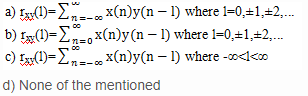Detailed Solution for Test: Discrete Time Signal Correlation - Question 2

Explanation: If any two signals x(n) and y(n) are real and finite energy signals, then the correlation between the two signals is known as cross correlation and its equation is given as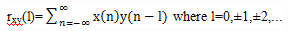Test: Discrete Time Signal Correlation - Question 3

### Which of the following relation is true?

Detailed Solution for Test: Discrete Time Signal Correlation - Question 3

Explanation: we know that, the correlation of two signals x(n) and y(n) is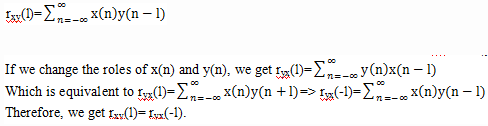Test: Discrete Time Signal Correlation - Question 4

What is the cross correlation sequence of the following sequences?
x(n)={….0,0,2,-1,3,7,1,2,-3,0,0….}
y(n)={….0,0,1,-1,2,-2,4,1,-2,5,0,0….}

Detailed Solution for Test: Discrete Time Signal Correlation - Question 4

Explanation: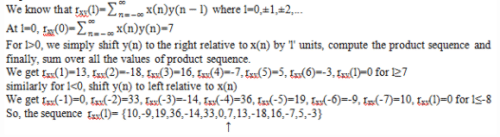Test: Discrete Time Signal Correlation - Question 5

Which of the following is the auto correlation of x(n)?

Detailed Solution for Test: Discrete Time Signal Correlation - Question 5

Explanation: We know that, the correlation of two signals x(n) and y(n) is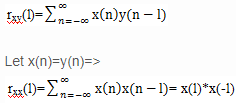Test: Discrete Time Signal Correlation - Question 6

What is the relation between cross correlation and auto correlation?

Detailed Solution for Test: Discrete Time Signal Correlation - Question 6

Explanation:
We know that, a2rxx(0)+b2ryy(0)+2abrxy(l) ≥0
=> (a/b)2rxx(0)+ryy(0)+2(a/b)rxy(l) ≥0
Since the quadratic is nonnegative, it follows that the discriminate of this quadratic must be non positive, that is 4[r2xy(l)- rxx(0) ryy(0)] ≤0 =>|rxy(l)|≤√(rxx(0).ryy(0)).

Test: Discrete Time Signal Correlation - Question 7

The normalized auto correlation ρxx(l) is defined as:

Detailed Solution for Test: Discrete Time Signal Correlation - Question 7

Explanation: If the signal involved in auto correlation is scaled, the shape of auto correlation does not change, only the amplitudes of auto correlation sequence are scaled accordingly. Since scaling is unimportant, it is often desirable, in practice, to normalize the auto correlation sequence to the range from -1 to 1. In the case of auto correlation sequence, we can simply divide by rxx (0). Thus the normalized auto correlation sequence is defined as ρxx(l)= (rxx (l))/(rxx (0)).

Test: Discrete Time Signal Correlation - Question 8

Auto correlation sequence is an even function.

Detailed Solution for Test: Discrete Time Signal Correlation - Question 8

Explanation: Let us consider a signal x(n) whose auto correlation is defined as rxx (l).
We know that, for auto correlation sequence rxx (l)=rxx (-l).
So, auto correlation sequence is an even sequence.

Test: Discrete Time Signal Correlation - Question 9

What is the auto correlation of the sequence x(n)=anu(n), 0<a<l?

Detailed Solution for Test: Discrete Time Signal Correlation - Question 9

Explanation: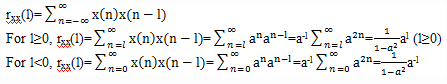Test: Discrete Time Signal Correlation - Question 10

If x(n) is the input signal of a system with impulse response h(n) and y(n) is the output signal, then the auto correlation of the signal y(n) is:

Detailed Solution for Test: Discrete Time Signal Correlation - Question 10

Explanation: ryy(l)=y(l)*y(-l)
=[h(l)*x(l)]*[h(-l)*x(-l)] =[h(l)*h(-l)]*[x(l)*x(-l)] =rhh(l)*rxx(l).

## Digital Signal Processing

3 videos|50 docs|54 tests
 Use Code STAYHOME200 and get INR 200 additional OFF Use Coupon Code
Information about Test: Discrete Time Signal Correlation Page
In this test you can find the Exam questions for Test: Discrete Time Signal Correlation solved & explained in the simplest way possible. Besides giving Questions and answers for Test: Discrete Time Signal Correlation, EduRev gives you an ample number of Online tests for practice

## Digital Signal Processing

3 videos|50 docs|54 tests